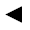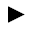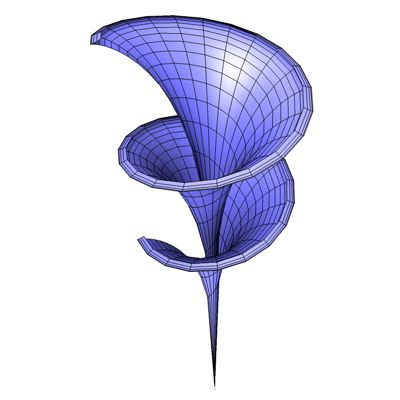﻿﻿﻿ math handbook calculator - Fractional Calculus Computer Algebra System software+++=﻿[back]

## 3.5. Dini's Surface

[next]The Dini Surface [ 2,7,11 ] is represented by the following equations.

 x = a cos(u) sin(v) 3-21 y = a sin(u) sin(v) 3-22 z = a [cos(v) + ln(tan(v/2))] + bu 3-23

The constants a and b determine the appearance of the figure.

To represent the area, the two parameters u and v can assume the following values ​​(domain of definition), for example.

 u is an element from the set of numbers [0, 4 pi] v is an element of the number set [0.01, 2]

The parameter v must not be zero, otherwise there will be an error in the calculation. The expression ln(tan(0.5 v)) is then not defined.Fig. 9